Latest Banking jobs   »

# Quantitative Aptitude Quiz For FCI Phase I 2022- 18th September

Directions (1-5) :- The line graph shows the no. of consumers of alcohol and cigarettes in a state in 5 different years. Study the graph carefully and answer the following questions.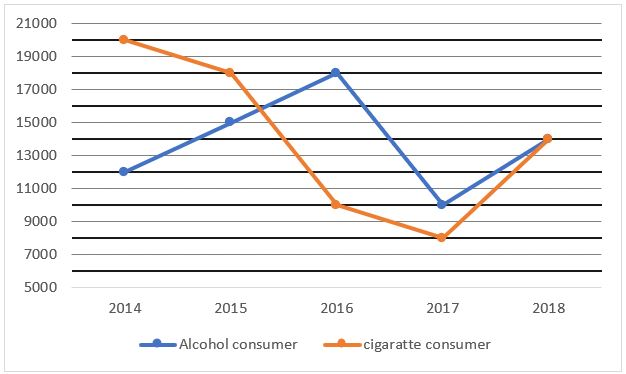Q1. Find the ratio between alcohol consumers in 2014 and 2016 together to the cigarette consumers in 2016 and 2017 together.
(a) 5 : 6
(b) 5 : 3
(c) 10 : 9
(d) 5 : 4
(e) 7 : 6

Q2. Find the no. of alcohol consumers increased or decreased in 2018 over 2014.
(a) 4000
(b) 3000
(c) 2000
(d) 5000
(e) 6000

Q3. What is the average no. of cigarette consumers over all the years?
(a) 12000
(b) 15000
(c) 13000
(d) 14000
(e) 16000

Q4. Cigarette consumers is what percent of alcohol consumers in 2015?
(a) 120%
(b) 83.33%
(c) 125%
(d) 150%
(e) 78%

Q5. Alcohol consumers in 2015 and 2017 together is what percent more/less than cigarette consumers in 2014 and 2016 together?
(a) 16.67%
(b) 23.25%
(c) 17.25%
(d) 20%
(e) 12.5%

Directions (6-10): – What approximate value should come in place of question mark (?) in the following questions?

Q6. 5012.97+987.23=?%of 3999
(a) 250
(b) 150
(c) 50
(d) 175
(e) 125

Q7. √1025+19.87% of 19.87=?²
(a) 5
(b) 4
(c) 3
(d) 6
(e) 2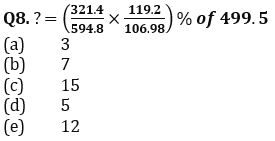Q9. 20%of150+11%of300=?²
(a) 5
(b) 8
(c) 4
(d) 2
(e) 9

Q10. 322.01-135.99+22.98=?
(a) 205
(b) 195
(c) 209
(d) 215
(e) 200

Directions (11 – 15): In each of these questions, two equations (I) and (II) are given. You have to solve both the equations and answer the following questions.
(a) x > y
(b) x < y
(c) x ≥ y
(d) x ≤ y
(e) x = y or no relation

Q11. I. 2x² – 11x + 15 = 0
II. 2y² – 13y + 20 = 0

Q12. I. 30/x²=11/x-1
II. y² – 7y + 12 = 0

Q13. I. x² – 84 = 112
II. y³ + 1845 = 5220

Q14. I. x² + 18x + 80 = 0
II. y² + 14y + 48 = 0

Q15. I. 18x – 12y = 9
II. 16y – 20x = 2

Solutions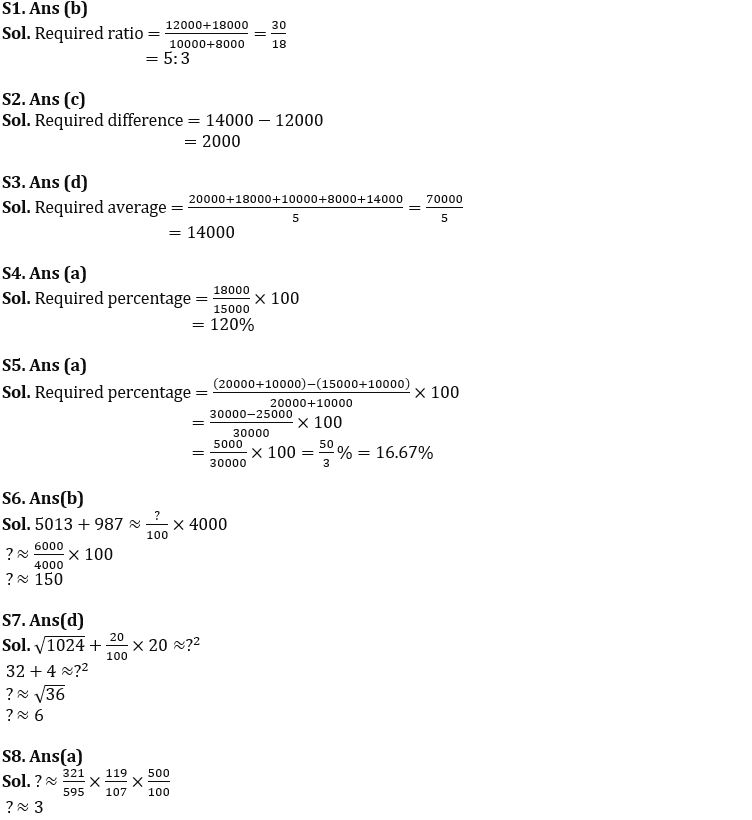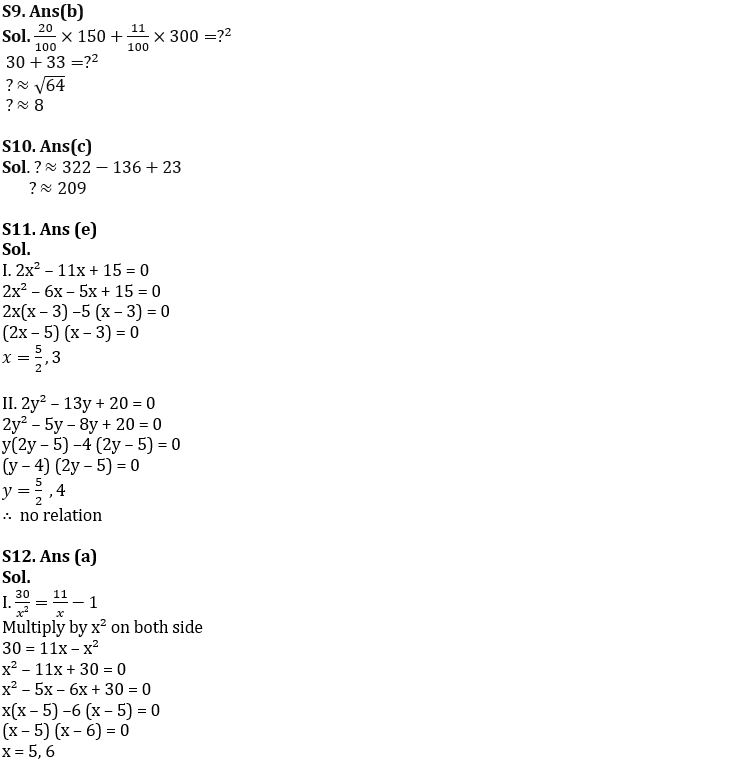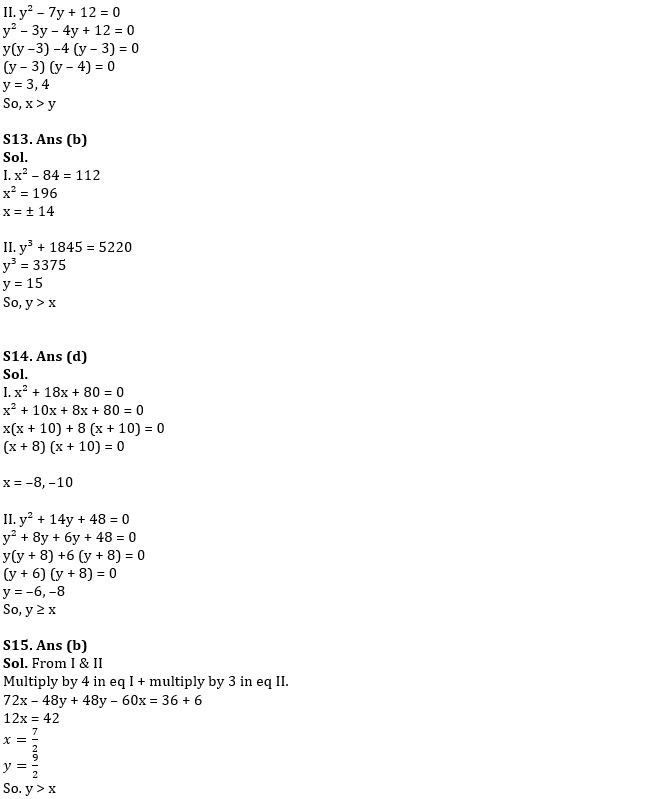#### Congratulations!Download Hindu Review of October 2021: Free PDF